# Rounding Off to the Nearest 10 on a Number Line

Rounding Off to the Nearest 10 on a Number Line• Rounding off a number to the nearest ten means to write down the closest value in the ten times table.
• To round off to the nearest ten using a number line, write down the two numbers in the ten times table that the given number is between.
• The smaller of these numbers will be the current number of tens that the given number contains and the larger of these numbers will be the next ten along.
• It helps to include every whole number on the number line.
• To find the nearest ten, simply count how many whole numbers each one is away from the given number.
• Numbers with 5 or more in the units digit will round up because they are past half way.
• Numbers with a 4 or less in the units digit will round down because they are before half way.
• In this example, 23 is nearer to 20 than it is to 30.

Mark the number on a number line, along with the two numbers in the ten times table that are either side of it.

The nearest ten is the closest of the two numbers in the ten times table.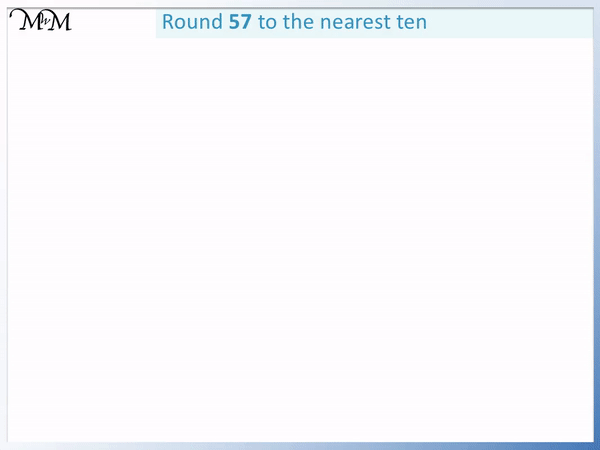• We mark 57 on the number line.
• 57 contains 5 tens and so it could round down to 50.
• The next ten up is 60 and so, 57 could round up to 60.
• We mark the whole numbers between 50 and 60.
• We count how far away 57 is from 50 and from 60 to see which ten is nearest.
• 57 is 7 places away from 50 and only 3 places away from 60.
• 57 is nearer to 60 and so, 57 rounds up to 60.
• We can see that 55 is the half way point on the number line and 57 is nearer to the 60 end of the line than it is to the 50 end.# How to Teach Rounding Off to the Nearest Ten using a Number Line

Rounding off a number to the nearest ten means to write down the multiple of ten that the given number is nearest to.

To teach rounding off to the nearest ten, it is helpful to introduce rounding using a number line. This helps to build an understanding of the relative sizes of each number and then to fully understand the process of rounding.

To round off to the nearest ten using a number line place the number on the number line and count how far away the closest multiple of ten is above or below this number. The closest number in the ten times table is the answer.

For each number there is a choice of whether to round down or to round up.

Rounding down to the nearest ten means to write down the number in the ten times table that is below the given number. This will be the number of tens that the given number contains.

Rounding up to the nearest ten means to write down the number in the ten times table that is above the given number. This will be the ten that is one more than the ten that the given number contains.

Below is the number 23. 23 contains ‘2’ tens, which is 20.

The choice is whether to round down to 20 or to round up to the next ten along, which is 30.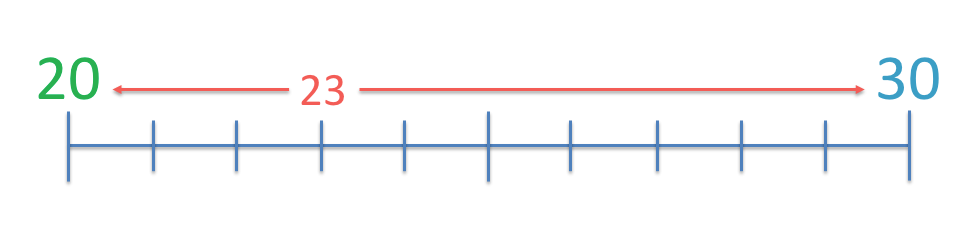On the number line, label the 23, 20 and 30.

When teaching rounding off using a number line, you will most likely be using a number line that is already completed with all whole numbers written on it. In this case we can simply find the number 23 and then look to the left and right to find the next numbers that have a ‘0’ digit in the units column.

Counting how far away 23 is from 20, we count: 22, 21 and 20.

23 is 3 whole numbers away from 20.

Counting how far away 23 is from 30, we count: 24, 25, 26 ,27, 28, 29 and 300.

23 is 7 whole numbers away from 30.

23 is nearer to 20 than it is to 30 and so, 23 rounds off downwards to 20.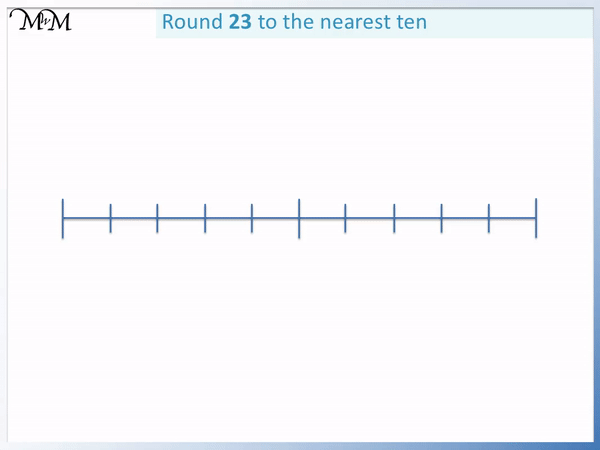We can see that 25 is the midway point between 20 and 30 and 23 is on the left of this point. We can see that because it is less than 25, it will round down.

We will now look at the example of 26. 26 contains a ‘2’ in the tens column. 2 tens are 20.

The choice is whether to round down to 20 or whether to round up to 30.Counting down to 20 we have: 25, 24, 23, 22, 21 and 20.

26 is 6 numbers larger than 20.

Counting up to 30 we have: 27, 28, 29 and 30.

26 is 4 numbers below 30.

26 is nearer to 30 than it is to 20 and so, 26 rounds up to 30.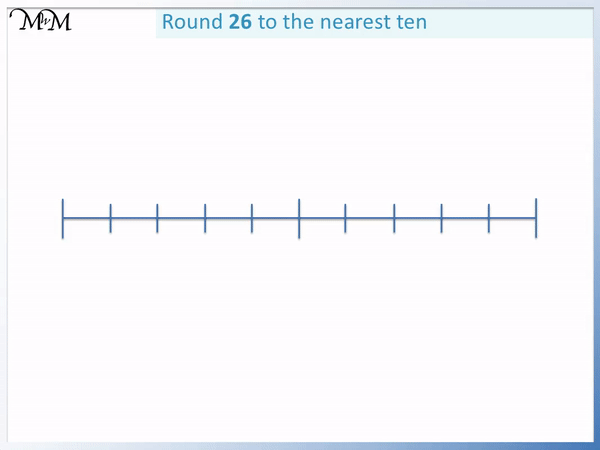Remember that 25 is the midway point between 20 and 30. We can see that 26 is nearer to 30 than 20 because it is on the right hand side of 25.

So we can see that on the number line, we can look at the midpoint between two tens and look at the position of the given number in comparison to this midpoint.The midpoint between any two tens will always by a number that ends in a 5 in the units digit.

When teaching rounding off using a number line, you can look at different tens and then mark the number that is directly in the middle to show this.

We need to decide how to round off 25, which is directly in between 20 and 30.

Do we decide to round down to 20 or round up to 30? We have to choose one of these multiples of ten.We decide to round 25 up.

The rule is that if a number ends in 5 or more then round it up and if a number ends in 4 or less, round it down.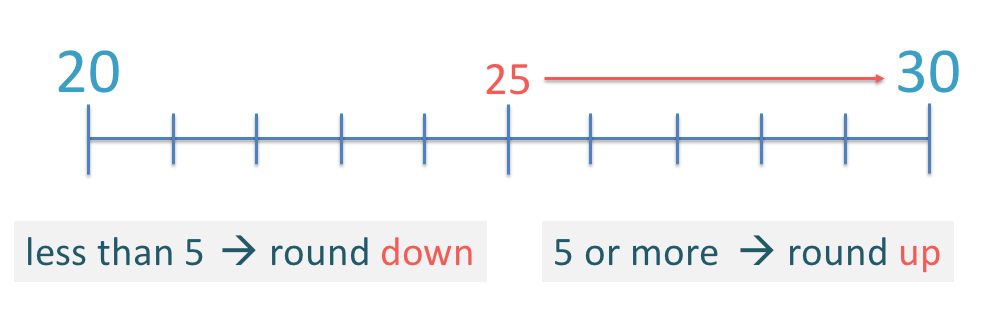The reason we round down numbers which end in 4 or less, is that they are on the left of the midpoint between two tens.

Any number ending in 6 or more is on the right of the midpoint and we round it up.

The reason we also round up numbers with 5 in the units digit is because as soon as we have any extra decimal digits attached, the number will move to the right of the midway point.

For example, 25.1 is now nearer to 30 than it is to 20.

By including 25 in the rule to round up means that we also correctly round up all numbers such as 25.1, 25.9 and 25.001.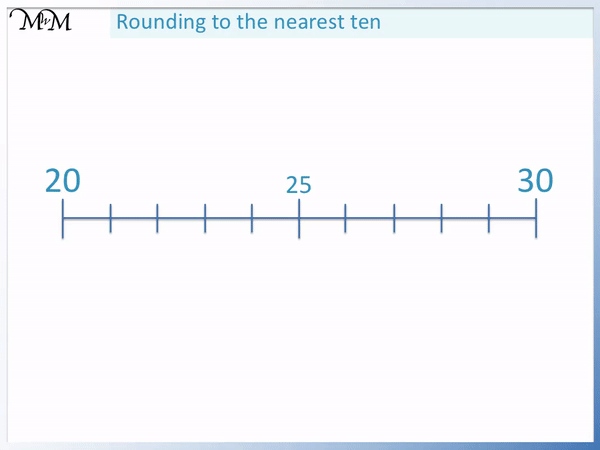Once we have learnt how to round off numbers using a number line, it is helpful to teach the link between the digits of a number, whilst still referring to the number line.

We will look at using the rounding off rule for the example of 57.The rounding off rule states that if the whole number ends in a 5 or more, then round up, otherwise round down.

57 ends in a ‘7’ digit.

We have the choice of whether to round down to 50 or up to 60.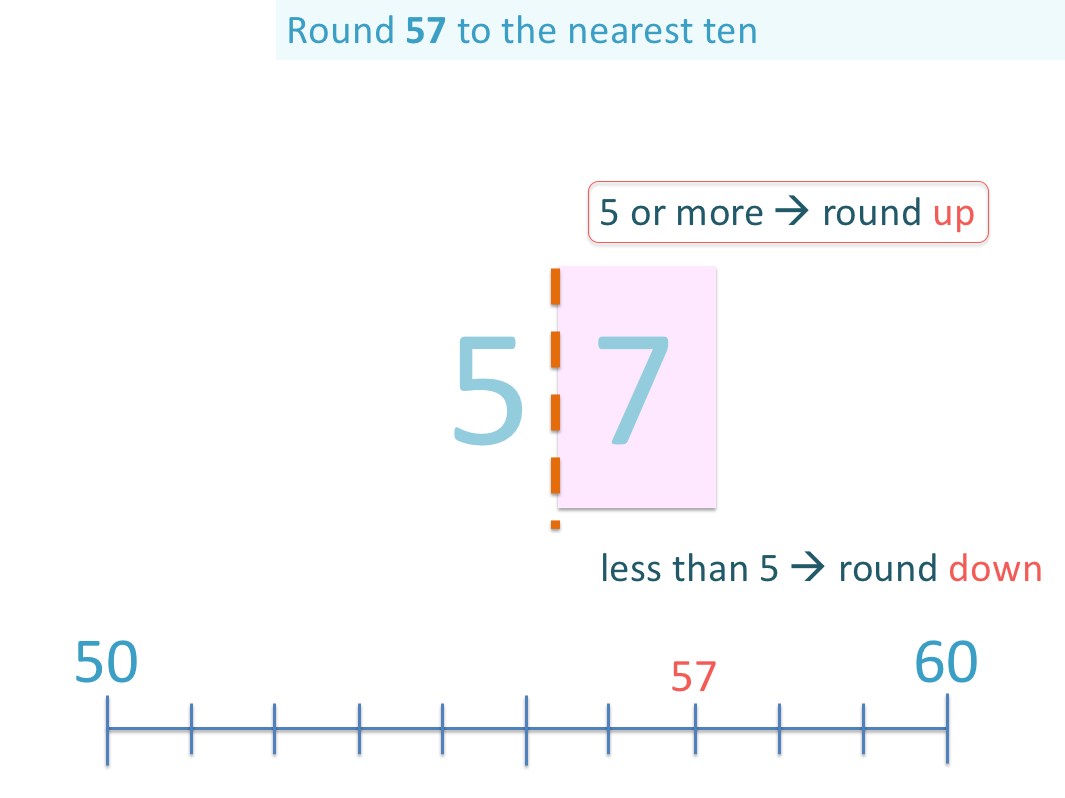Since 7 is 5 or more it rounds up.

57 rounds off to 60 when written to the nearest ten.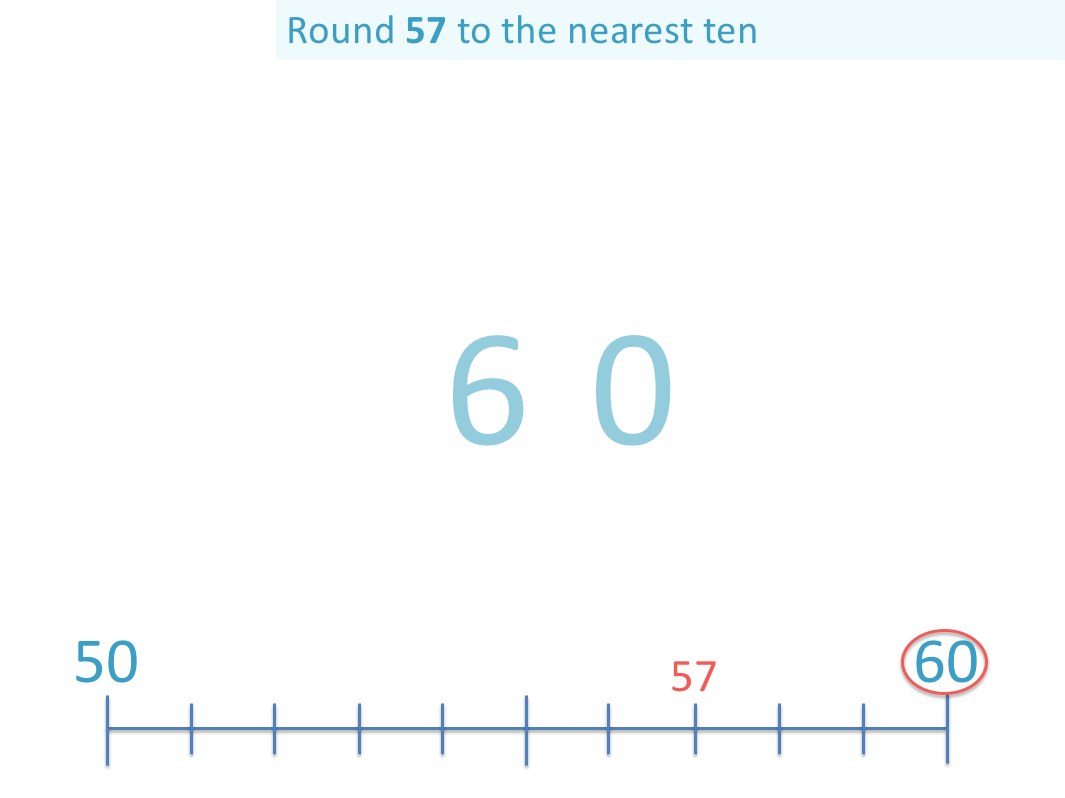57 is nearer to 60 than it is to 50.When first teaching this rounding off rule, remember to refer to the number line to build the understanding of why the rule works.

We will look at the example of rounding off 83 below.We look at the digit in the units column, which is a ‘3’.

The rounding off rule says that if this digit is 4 or less, then round down.Since the number ends with a 3 in the units column, it rounds down.83 rounds off to 80.

83 is nearer to 80 than it is to 90 because it is left of the midway point of 85.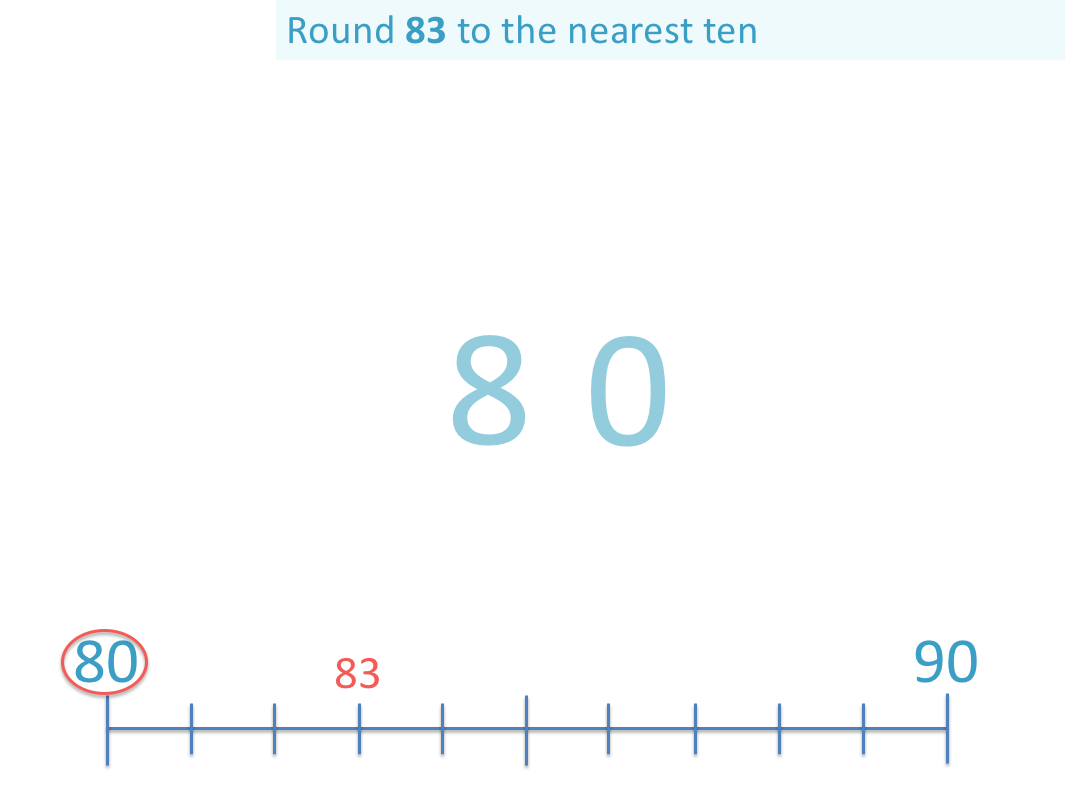Here is an example of rounding 95 off to the nearest ten.On a number line, 95 is directly in between 90 and 100.

90 and 100 are the first two numbers either side of 95 that are in the ten times table. 100 is 10 lots of 10.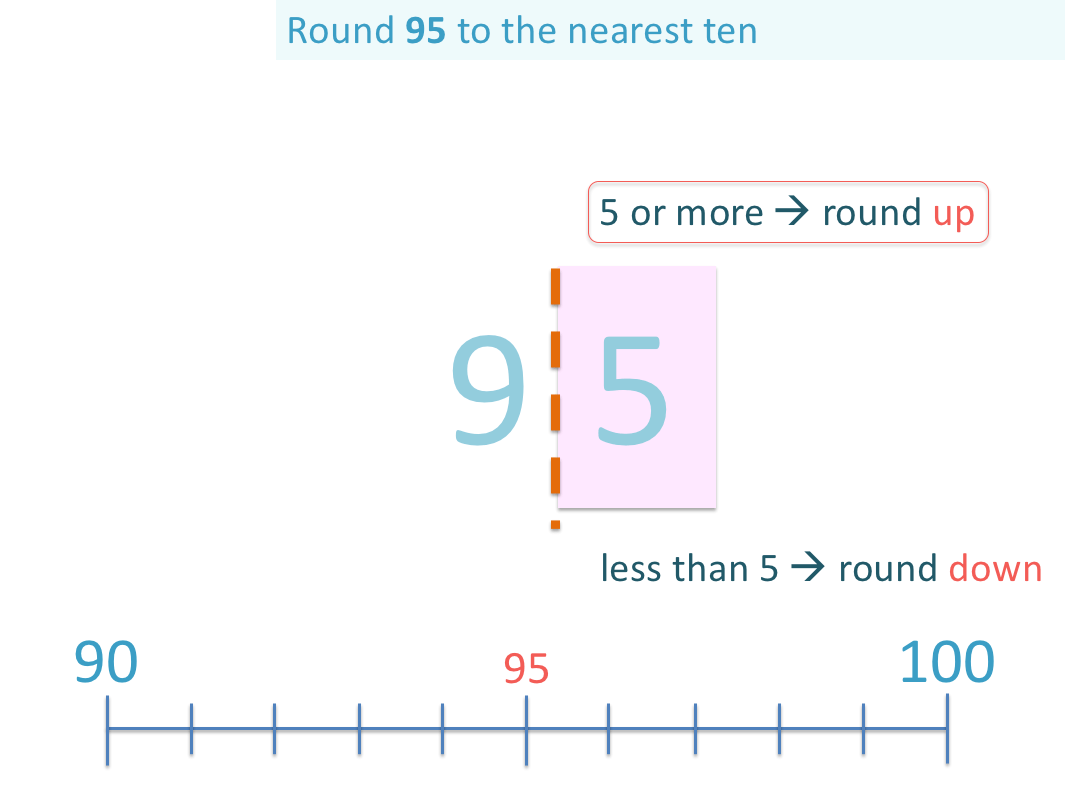Remember that the rounding off rule says that any number with a 5 or more in the units column will round up.

Even though 95 is directly in between 90 and 100, we round it up.95 rounds off to 100 when written to the nearest 10.Now try our lesson on Rounding Whole Numbers to the Nearest Ten where we learn how to round any whole number to the nearest ten.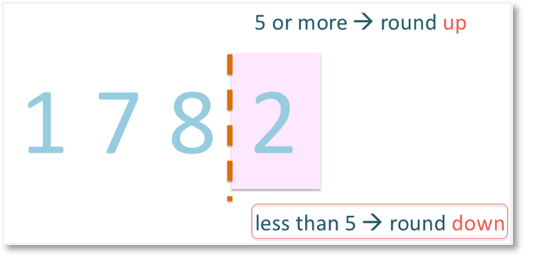error: Content is protected !!